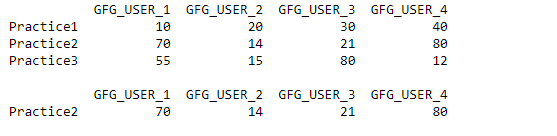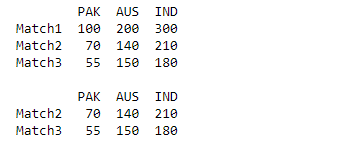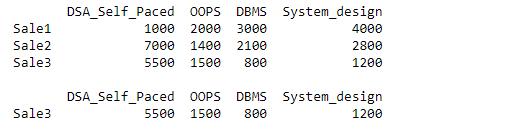Related Articles

# Selecting with complex criteria using query method in Pandas

• Last Updated : 25 Oct, 2020

In this article, let’s discuss how to select complex criteria using the Query() method in Pandas. In pandas for Selecting with complex criteria using the query method, first, we create data frames with the help of pandas.Dataframe() and store it one variable and then with the help of query() method we can select complex criteria. With the help of pandas.Dataframe.loc() we can find details of the data frame by passing the index of the data frame.
Example 1:

## Python3

 `import` `pandas as pd`` ` `df ``=` `pd.DataFrame([[``10``, ``20``, ``30``, ``40``], [``70``, ``14``, ``21``, ``80``], ``                   ``[``55``, ``15``, ``80``, ``12``]],``                   ` `                  ``columns``=``[``'GFG_USER_1'``, ``'GFG_USER_2'``,``                           ``'GFG_USER_3'``, ``'GFG_USER_4'``],``                   ` `                  ``index``=``[``'Practice1'``, ``'Practice2'``, ``'Practice3'``])`` ` `print``(df, ``"\n"``)`` ` `# Filter data using query method``df1 ``=` `df.loc[df.query(``    ``'GFG_USER_1 <``=` `80` `& GFG_USER_2 > ``10` `& \``    ``GFG_USER_3 < ``50` `&  GFG_USER_4 ``=``=` `80``').index]`` ` `print``(df1)`

Output:Example 2:

## Python3

 `import` `pandas as pd`` ` `df ``=` `pd.DataFrame([[``100``, ``200``, ``300``], [``70``, ``140``, ``210``], ``                   ``[``55``, ``150``, ``180``]],``                   ` `                  ``columns``=``[``'PAK'``, ``'AUS'``, ``'IND'``],``                   ` `                  ``index``=``[``'Match1'``, ``'Match2'``, ``'Match3'``])`` ` `print``(df, ``"\n"``)`` ` `# Filter data using query method``df1 ``=` `df.loc[df.query(``'PAK <= 80 & AUS > 100 & IND < 250'``).index]`` ` `print``(df1)`

Output:Example 3:

## Python3

 `import` `pandas as pd`` ` `df ``=` `pd.DataFrame([[``1000``, ``2000``, ``3000``, ``4000``], [``7000``, ``1400``, ``2100``, ``2800``], ``                   ``[``5500``, ``1500``, ``800``, ``1200``]],``                   ` `                  ``columns``=``[``'DSA_Self_Paced'``, ``'OOPS'``, ``'DBMS'``, ``'System_design'``],``                   ` `                  ``index``=``[``'Sale1'``, ``'Sale2'``, ``'Sale3'``])`` ` `print``(df, ``"\n"``)`` ` `# Filter data using query method``df1 ``=` `df.loc[df.query(``    ``'DSA_Self_Paced <``=` `6000` `& OOPS > ``1400` `& DBMS < ``1500` `\``    ``&  System_design ``=``=` `1200``').index]`` ` `print``(df1)`

Output:Attention geek! Strengthen your foundations with the Python Programming Foundation Course and learn the basics.

To begin with, your interview preparations Enhance your Data Structures concepts with the Python DS Course. And to begin with your Machine Learning Journey, join the Machine Learning – Basic Level Course

My Personal Notes arrow_drop_up